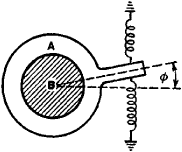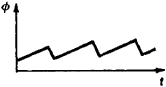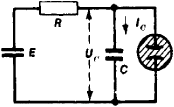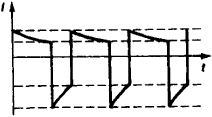# Relaxation Oscillations

## relaxation oscillations

[‚rē‚lak′sā·shən ‚äs·ə‚lā·shənz]
(physics)
Oscillations having a sawtooth waveform in which the displacement increases to a certain value and then drops back to zero, after which the cycle is repeated.
McGraw-Hill Dictionary of Scientific & Technical Terms, 6E, Copyright © 2003 by The McGraw-Hill Companies, Inc.
The following article is from The Great Soviet Encyclopedia (1979). It might be outdated or ideologically biased.

## Relaxation Oscillations

self-maintained oscillations that arise in systems in which dissipative forces play an important role. Examples of such forces are external or internal friction in mechanical systems and resistance in electrical systems. Because these forces dissipate energy, the energy stored in one of two (or more) storage elements in such an oscillating system does not pass entirely to the other storage element, as it does in systems that perform harmonic oscillations, but is partially dissipated in the system into thermal energy.

Since relaxation oscillations, like any self-maintained oscillations, can occur only in nonlinear systems, the study of relaxation oscillations requires the application of the nonlinear theory of oscillations. It is characteristic of relaxation systems that when the energy source is disconnected, the oscillatory motion cannot occur. Suppose one energy-consuming parameter is of primary importance in the system—for example, the capacitance when the inductance is negligibly small or the elasticity when the mass is negligibly small. Each period of the relaxation oscillations can then be divided into several sharply delineated stages that correspond to slow and fast changes in the state of the system in which the relaxation oscillations occur. Consequently, relaxation oscillations in such degenerate systems can be regarded as discontinuous oscillations.Figure 1. Mechanical relaxation system

A simple example of a mechanical system that produces relaxation oscillations is a shoe that is pressed against a rotating shaft B and is held in position by springs (Figure 1). When the shaft rotates, friction causes the shoe to be pulled along by the shaft until the moment of the elastic forces of the springs becomes equal to the maximum possible moment of the frictional forces. The shoe then begins slipping along the shaft in the opposite direction. As the shoe slips, the relative velocity between the shoe and shaft increases, the frictional force decreases, and the shoe returns to its initial position. As the shoe approaches the equilibrium position, however, the elastic force of the springs decreases, and the shaft once again catches hold of the shoe and draws it along. The process is then repeated (Figure 2).Figure 2. Graph of variation in the shoe’s angle of rotation with time: (Φ) angle of rotation, (t) time

Mechanical relaxation oscillations are encountered in various mechanisms. An example is a brake shoe. For a mechanism to perform relaxation oscillations, the friction must be sufficiently great, and the magnitude of the friction must decrease (at least in some region) as the relative rate of motion between the surfaces affected by the frictional forces increases.

A simple example of electrical relaxation oscillations are the oscillations that arise under certain conditions in a circuit withFigure 3. Electrical relaxation system

a gas-discharge tube. In such a tube, the discharge is established at a breakdown voltage Ub and is extinguished at a lower voltage Ue, which is called the extinction voltage. In the circuit shown in Figure 3, the capacitance C is periodically charged from the current source E through the resistance R up to the breakdown voltage of the tube. At this time, the discharge is established, and the capacitance discharges rapidly through the tube until the extinction voltage is reached. The discharge is then extinguished, and the process begins anew. In each period of these relaxation oscillations, two slow changes in the current I occur during the charging and discharging of the capacitance; two abrupt changes occur in the current Ic when the discharge is established and extinguished (Figure 4).Figure 4. Graph of variation in current with time in a circuit with a gas-discharge tube: (I) current, (t) time

The mechanism of occurrence of relaxation oscillations can be studied in a simplified manner by disregarding the parameters of the system that affect the character of the abrupt or fast motions. The techniques of the nonlinear theory of oscillations permit both the slow and fast motions to be investigated without disregarding the parameters that essentially determine the nature of the fast motions and without resorting to special postulates as to the character of the fast motions. Depending on the properties of the system, relaxation oscillations can take on a great variety of forms—ranging from almost harmonic oscillations to discontinuous and pulse-type oscillations.

Electrical relaxation oscillations are used widely in such branches of electronics as measurement technology, remote control, and automation. Various relaxation-oscillator circuits are used for the generation of relaxation oscillations. Examples are blocking oscillators, multivibrators, and RC-oscillators.

### REFERENCES

Andronov, A. A., A. A. Vitt, and S. E. Khaikin. Teoriia kolebanii, 2nd ed. Moscow, 1959. Chapters 4 and 9.
Meerovich, L. A., and L. G. Zelichenko. Impul’snaia tekhnika, 2nd ed. Moscow, 1954. Chapters 14–15.
References in periodicals archive ?
The limit cycles occurring in singularly perturbed Lienard systems are relaxation oscillations, characterized by the presence of phases in the cycle with different time scales: A phase of slow change is followed by a short phase of rapid change where the system jumps to the next stage of slow variation.
In this paper, we will extend the asymptotic analysis method of Eckhaus  to study the birth of relaxation oscillations in the following singularly perturbed generalized Lienard system,
Relaxation oscillations are described by two alternating processes on different time scales; a long relaxation period during which the system structure approaches an equilibrium point, exchanging with a short impulsive period in which the equilibrium point transfers.
On the problem of control of relaxation oscillations of a "singing" flame.
Here slow-fast cycle is not periodic orbit of system (2), but a limit periodic set as [epsilon] approaches 0 and the limit cycles that are close to slow-fast cycle are called relaxation oscillations. In 2007, Dumortier et al.
So there exists ([u.sub.0], [v.sub.0], [w.sub.0]) which satisfies the conditions in the third part of Theorem 1, and from conclusions of Theorem 1, we get that system (32) has three hyperbolic relaxation oscillations near canard cycle [[GAMMA].sub.UVW], where U = [F.sub.0]([u.sub.0]), V = [F.sub.0](v([u.sub.0])), W = [F.sub.0](w([u.sub.0])), [u.sub.0] [member of] (-1, 0).
When coupling is weak, the model exhibits low-frequency antiphase relaxation oscillations with each species alternatively taking the dominant role.
As seen in this figure, the rise time is exactly equal to the turnon delay and the time of the relaxation oscillations which is less than 20 psec.
The dynamics of this so-called "spurt" regime can be described quantitatively by way of the theory of relaxation oscillations in terms of pressure and flow rate as reported by Molenaar et al.
When [f.sub.m] increases beyond [f.sub.r], the electron and photon fields tend to become more and more out of phase, resulting in damping of the relaxation oscillations and reduction in the IM response.
Van der Pol equation for relaxation oscillation. In Contribution to the theory of nonlinear oscillation II.

Site: Follow: Share:
Open / Close